## The Isoperimetric Inequality

Given a rope of length L, say 100 meters, what is the largest closed area you can make?
If we try a square, each side has to be L/4 (25 meters), and we'll end up with an area of (L/4)^2 = 625 square meters. If we however try a circle, the circumference is L=2πR, where R is the radius. Thus R = L/(2π), and the area is π(L/2π)^2 = L^2/(4π) = 796 square meters, which is better than the square.

But there's plenty more shapes to try, in fact, infinitely many. So which should we pick to maximize the area?
The ancients knew the answer but could not prove it: it's the circle. The proof of this is surprisingly simple using calculus. Let L be the length of the rope, and let A be the enclosed area.

Note that for a circle, the relationship between A and L is L=2πR and A=πR^2, where R is the radius, so that: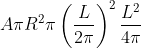Given the belief that the circle gives the largest area, we expect that for any shape,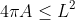This is known as the isoperimetric inequality.

## Hurwitz's Proof

Assume that the curve (x(t),y(t)) is parametrized so that it does one full revolution when t goes from 0 to π. The arc length is defined by s = Lt/(2π). Note that when t=0, s=0, and when t=2π, s=L.

We then have: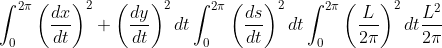As for the area, we use Green's theorem: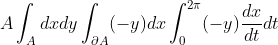Now, simply take the difference we want to show is always non-negative:We want to conclude that this expression is non-negative for any curve. The first integral is clearly non-negative, since its integrand is a square. As for the second integral, the result is not obvious until one consider its Fourier series (this is called Wirtinger's inequality):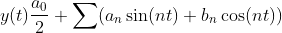As we will see soon, for this to work, we must place the curve in coordinates such that: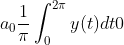This can always be done, as the placement of the curve is arbitrary: if the integral of y(t) is V, then the translated function y(t)-V/(2π) will have integral 0.

Next, differentiate y(t) to obtain: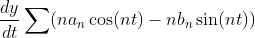Then, by Parseval's identity: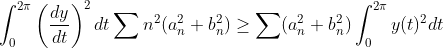which concludes the proof. (The requirement a0=0 is to ensure that the last inequality holds for n=0).
The inequality thus obtained is known as the isoperimetric inequality:## Only the Circle Yields Maximum Area

As we saw in the beginning, the circle turns the inequality into an equality, thus yielding the maximum possible area. But this still leaves open the possibility that other shapes may be equally good. We can show that only the circle yields max area by considering when the inequality becomes an equality: precisely when the two integrals are zero.

Starting with the second integral, the inequality was obtained from comparing the square sums after applying Parseval's identity. To have equality, the terms in those sums must be 0 except when n=1, which means that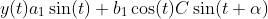The first integral must also be zero, which means that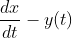Integrating, we get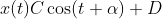So indeed we must have a circle centered at (D,0).

The fact that the circle must be centered around y=0 is due to our previous choice of fixing the integral of y(t) over one period. The center of mass must be such that equally much is positive and negative, which for a circle clearly means fixing it around y=0.

## How general is this proof?

The use of Green's theorem requires that we deal with a curve (x(t),y(t)) that has a continuous derivative except in finitely many points (piecewise smooth). Piecewise smoothness is also enough to ensure that the Fourier series can be differentiated term by term. Any area you can physically construct will certainly satisfy these conditions, as will anything you draw with a pen, since it is impossible to create or draw infinitely many edged points.